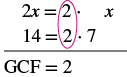## Finding the Greatest Common Factor of a Polynomial

### Learning Outcomes

• Factor the greatest common factor from a polynomial

Just like in arithmetic, where it is sometimes useful to represent a number in factored form (for example, $12$ as $2\cdot 6\text{ or }3\cdot 4$) in algebra it can be useful to represent a polynomial in factored form. One way to do this is by finding the greatest common factor of all the terms. Remember that you can multiply a polynomial by a monomial as follows:

$\begin{array}{ccc}\hfill 2\left(x + 7\right)&\text{factors}\hfill \\ \hfill 2\cdot x + 2\cdot 7\hfill \\ \hfill 2x + 14&\text{product}\hfill \end{array}$

Here, we will start with a product, like $2x+14$, and end with its factors, $2\left(x+7\right)$. To do this we apply the Distributive Property “in reverse”.

### Distributive Property

If $a,b,c$ are real numbers, then

$a\left(b+c\right)=ab+ac\text{ and }ab+ac=a\left(b+c\right)$

The form on the left is used to multiply. The form on the right is used to factor.

So how do we use the Distributive Property to factor a polynomial? We find the GCF of all the terms and write the polynomial as a product!

### example

Factor: $2x+14$

Solution

 Step 1: Find the GCF of all the terms of the polynomial. Find the GCF of $2x$ and $14$.Step 2: Rewrite each term as a product using the GCF. Rewrite $2x$ and $14$ as products of their GCF, $2$. $2x=2\cdot x$ $14=2\cdot 7$ $2x+14$ $\color{red}{2}\cdot x+\color{red}{2}\cdot7$ Step 3: Use the Distributive Property ‘in reverse’ to factor the expression. $2\left(x+7\right)$ Step 4: Check by multiplying the factors. Check: $2(x+7)$ $2\cdot{x}+2\cdot{7}$ $2x+14\quad\checkmark$

### try it

Notice that in the example, we used the word factor as both a noun and a verb:

$\begin{array}{cccc}\text{Noun}\hfill & & & 7\text{ is a factor of }14\hfill \\ \text{Verb}\hfill & & & \text{factor }2\text{ from }2x+14\hfill \end{array}$

### Factor the greatest common factor from a polynomial

1. Find the GCF of all the terms of the polynomial.
2. Rewrite each term as a product using the GCF.
3. Use the Distributive Property ‘in reverse’ to factor the expression.
4. Check by multiplying the factors.

### example

Factor: $3a+3$

### try it

The expressions in the next example have several factors in common. Remember to write the GCF as the product of all the common factors.

### example

Factor: $12x - 60$

### try it

Watch the following video to see more examples of factoring the GCF from a binomial.

Now we’ll factor the greatest common factor from a trinomial. We start by finding the GCF of all three terms.

### example

Factor: $3{y}^{2}+6y+9$

### try it

In the next example, we factor a variable from a binomial.

### example

Factor: $6{x}^{2}+5x$

### try it

When there are several common factors, as we’ll see in the next two examples, good organization and neat work helps!

### example

Factor: $4{x}^{3}-20{x}^{2}$

### example

Factor: $21{y}^{2}+35y$

### example

Factor: $14{x}^{3}+8{x}^{2}-10x$

### try it

When the leading coefficient, the coefficient of the first term, is negative, we factor the negative out as part of the GCF.

### example

Factor: $-9y - 27$

### try it

Pay close attention to the signs of the terms in the next example.

### example

Factor: $-4{a}^{2}+16a$